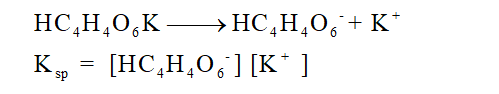# Using the general format of the Ksp equation figure out the molarity of both [K+] and [HC4H4O6-] if the theoretical Ksp= 3.8*10^-4 (Ksp= [K+][KC4H4O6-]

Question
2 views

Using the general format of the Ksp equation figure out the molarity of both [K+] and [HC4H4O6-] if the theoretical Ksp= 3.8*10^-4 (Ksp= [K+][KC4H4O6-]

check_circle

Step 1

All known compounds can be dissolve in certain solvents whereas they remain insoluble in other solvents. The solubility of any substance can be determined with the help of solubility product.

Step 2

Solubility product of any compounds can be defined as the mathematical product of dissolved ion concentrations of any compound raised to the power of their stoichiometric coefficients. It is represented as Ksp.

Step 3

The ionization of given potassium salt can be written in the given way:Hence the Ksp expression must be:...

### Want to see the full answer?

See Solution

#### Want to see this answer and more?

Solutions are written by subject experts who are available 24/7. Questions are typically answered within 1 hour.*

See Solution
*Response times may vary by subject and question.
Tagged in